CBSE Class 10 Sample Paper for 2022 Boards - Maths Standard [Term 2]

Class 10
Solutions of Sample Papers for Class 10 Boards

## ii) After 10 minutes, the guard observed that the boat was approaching the tower and its distance from tower is reduced by 240(√3 - 1) m. He immediately raised the alarm. What was the new angle of depression of the boat from the top of the observation tower?

This question is similar to Ex 9.1, 13 Chapter 9 Class 10 - Some Applications of Trigonometry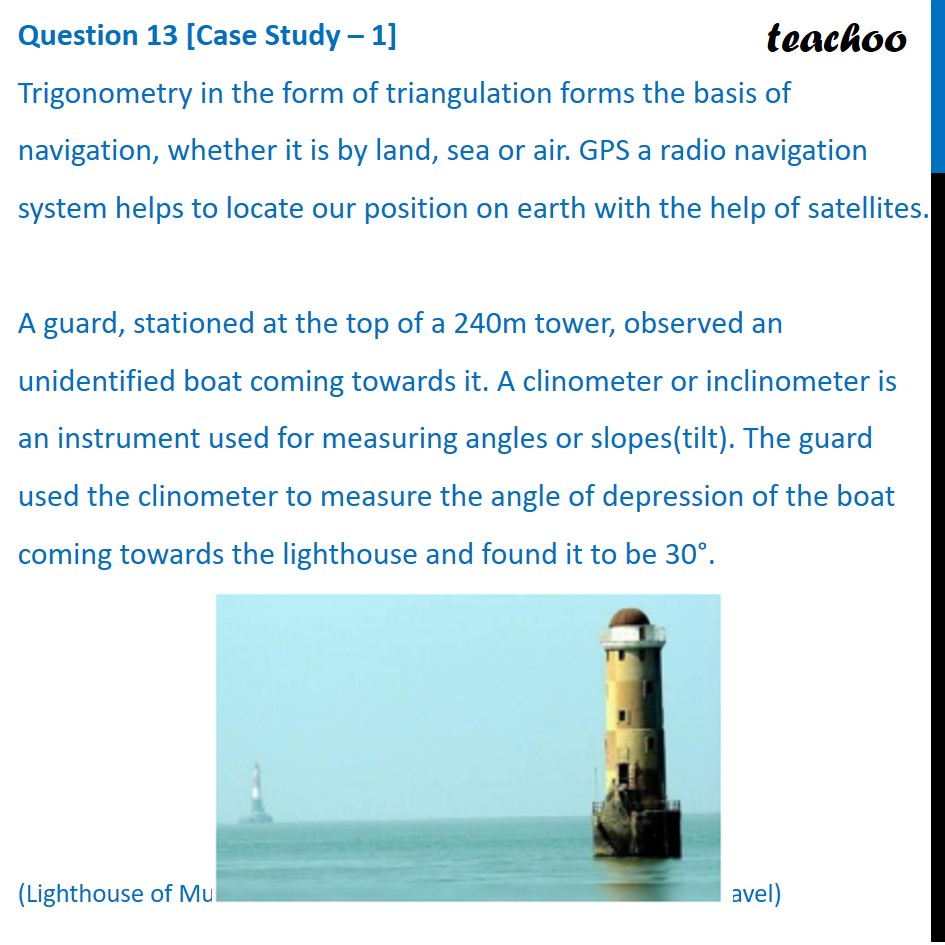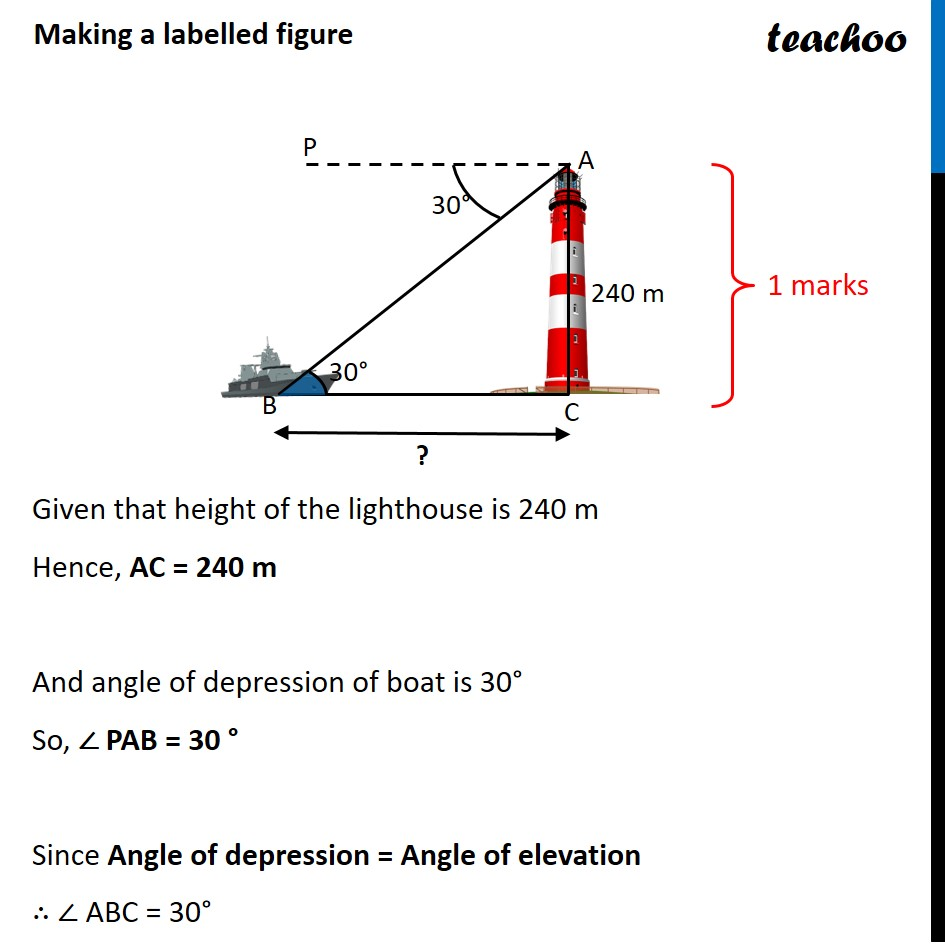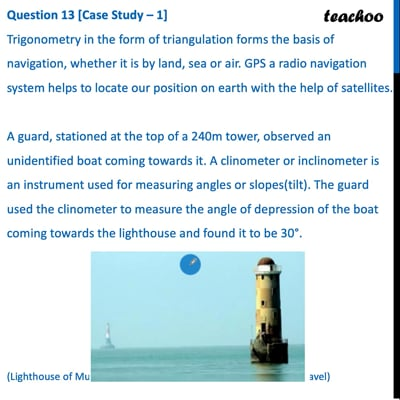This video is only available for Teachoo black users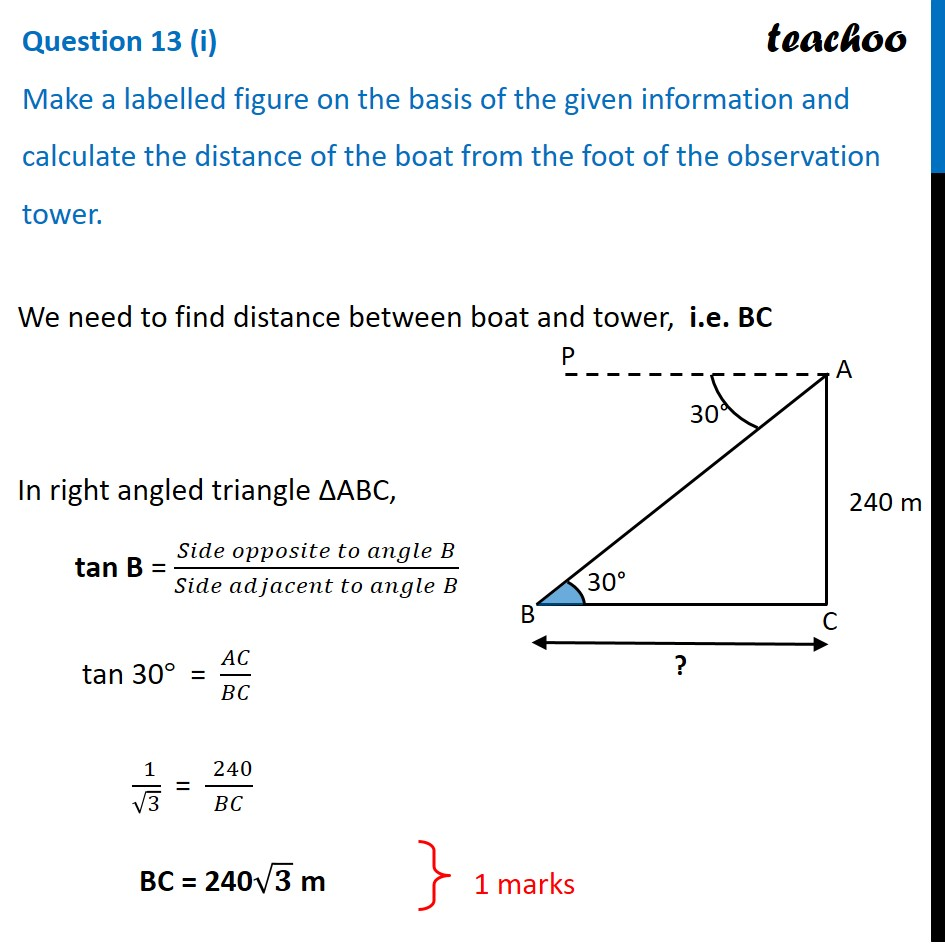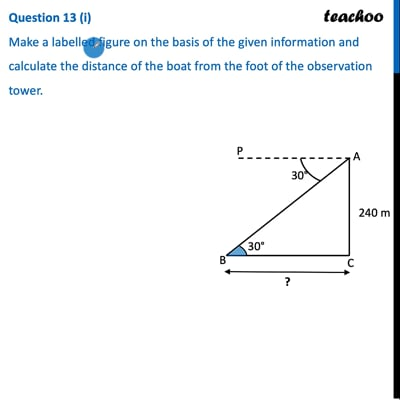This video is only available for Teachoo black users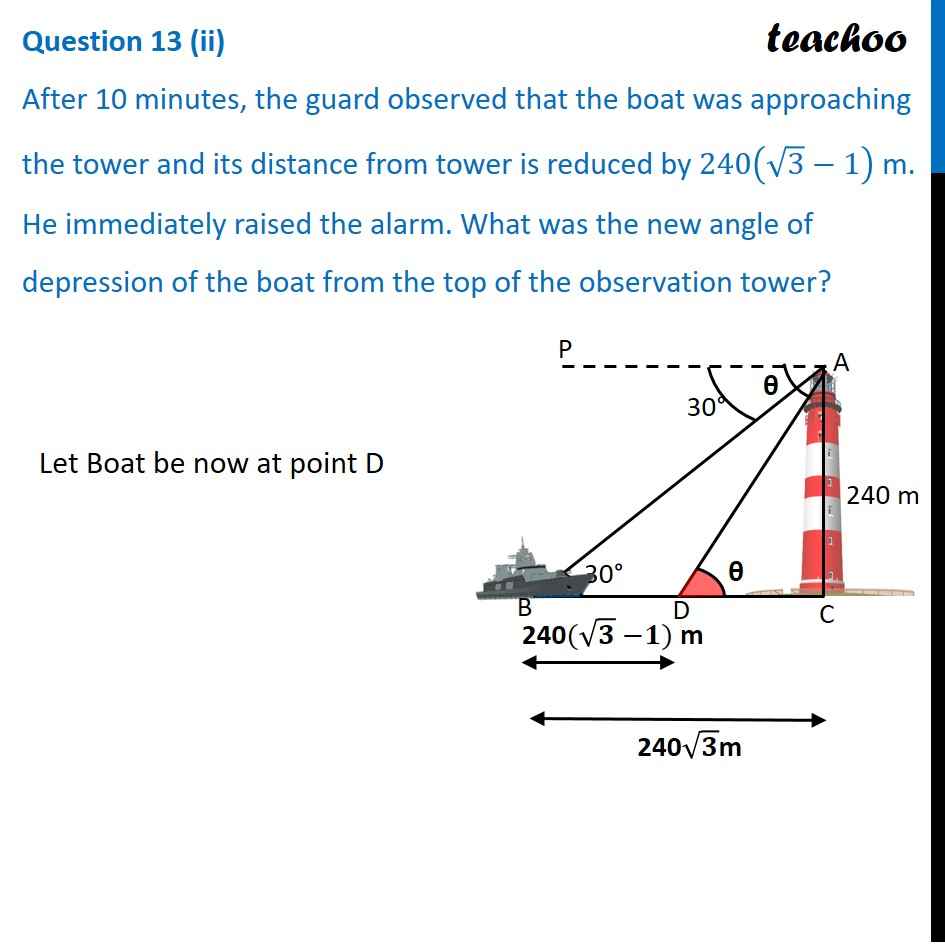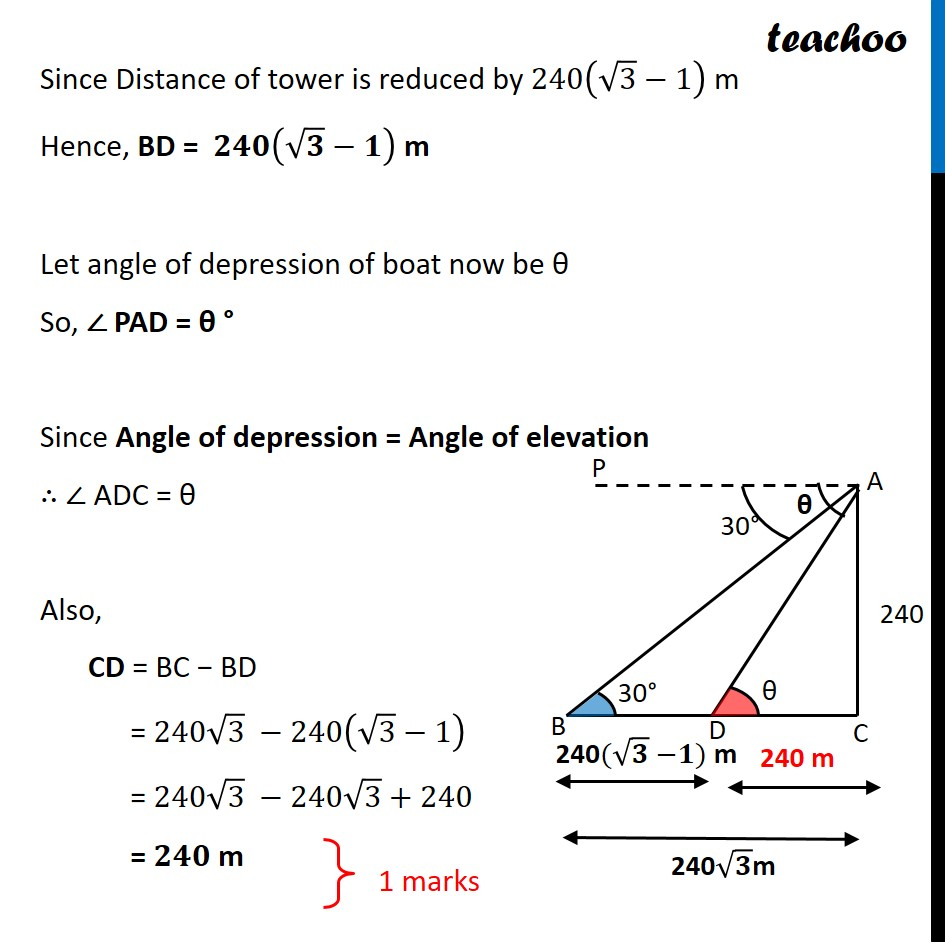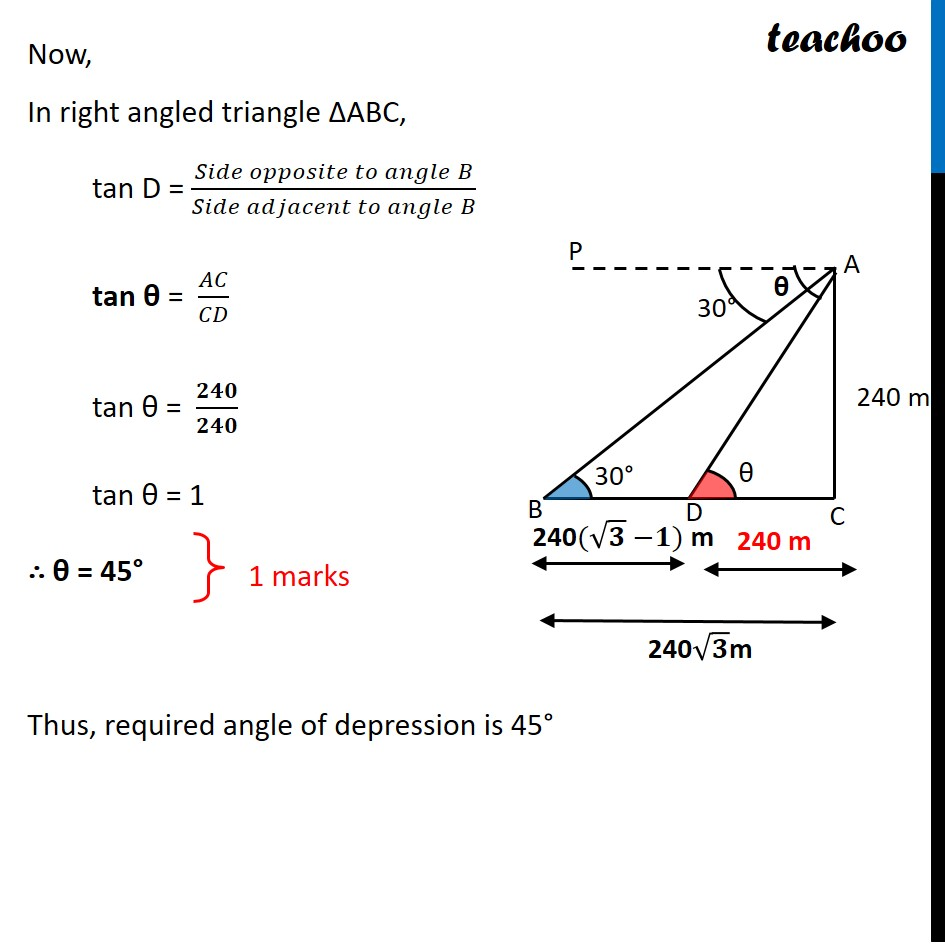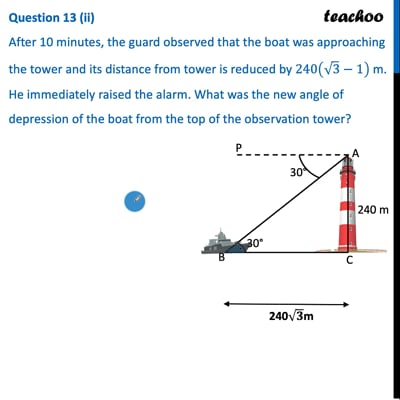This video is only available for Teachoo black users

### Transcript

Trigonometry in the form of triangulation forms the basis of navigation, whether it is by land, sea or air. GPS a radio navigation system helps to locate our position on earth with the help of satellites. A guard, stationed at the top of a 240m tower, observed an unidentified boat coming towards it. A clinometer or inclinometer is an instrument used fo measuring angles or slopes(tilt). The guard used the clinometer to measure the angle of depression of the boat coming towards the lighthouse and found it to be 30°. (Lighthouse of Mumbai Harbour. Picture credits - Times of India Travel) i) Make a labelled figure on the basis of the given information and calculate the distance of the boat from the foot of the observation tower. ii) After 10 minutes, the guard observed that the boat was approaching the tower and its distance from tower is reduced by 240(√3 - 1) m. He immediately raised the alarm. What was the new angle of depression of the boat from the top of the observation tower? Making a labelled figure Given that height of the lighthouse is 240 m Hence, AC = 240 m And angle of depression of boat is 30° So, ∠ PAB = 30 ° Since Angle of depression = Angle of elevation ∴ ∠ ABC = 30° Question 13 (i) Make a labelled figure on the basis of the given information and calculate the distance of the boat from the foot of the observation tower. We need to find distance between boat and tower, i.e. BC In right angled triangle ΔABC, tan B = (𝑆𝑖𝑑𝑒 𝑜𝑝𝑝𝑜𝑠𝑖𝑡𝑒 𝑡𝑜 𝑎𝑛𝑔𝑙𝑒" " 𝐵)/(𝑆𝑖𝑑𝑒 𝑎𝑑𝑗𝑎𝑐𝑒𝑛𝑡 𝑡𝑜 𝑎𝑛𝑔𝑙𝑒" " 𝐵) tan 30° = 𝐴𝐶/𝐵𝐶 (" " 1)/√3 = (" " 240)/𝐵𝐶 BC = 240√𝟑 m Question 13 (ii) After 10 minutes, the guard observed that the boat was approaching the tower and its distance from tower is reduced by 240(√3−1) m. He immediately raised the alarm. What was the new angle of depression of the boat from the top of the observation tower? Let Boat be now at point D Since Distance of tower is reduced by 240(√3−1) m Hence, BD = 𝟐𝟒𝟎(√𝟑−𝟏) m Let angle of depression of boat now be θ So, ∠ PAD = θ ° Since Angle of depression = Angle of elevation ∴ ∠ ADC = θ Also, CD = BC − BD = 240√3 −240(√3−1) = 240√3 −240√3+240 = 𝟐𝟒𝟎 m Now, In right angled triangle ΔABC, tan D = (𝑆𝑖𝑑𝑒 𝑜𝑝𝑝𝑜𝑠𝑖𝑡𝑒 𝑡𝑜 𝑎𝑛𝑔𝑙𝑒" " 𝐵)/(𝑆𝑖𝑑𝑒 𝑎𝑑𝑗𝑎𝑐𝑒𝑛𝑡 𝑡𝑜 𝑎𝑛𝑔𝑙𝑒" " 𝐵) tan θ = 𝐴𝐶/𝐶𝐷 tan θ = 𝟐𝟒𝟎/𝟐𝟒𝟎 tan θ = 1 ∴ θ = 45° Thus, required angle of depression is 45°